# Conditioning and error analysis¶

In this lab we will explore condition numbers and error analysis. To do this, we will need to be able to generate random points.

## Random Point Generation¶

A histogram counts the number of points in a vector that are inside bins, and can be used to plot the distribution that random points are drawn from. For example, randn generates normal distributed random number, distributed like

$${e^{-{x^2 \over 2}} \over \sqrt{2π}}:$$
In :
using PyPlot

plt[:hist](randn(10000));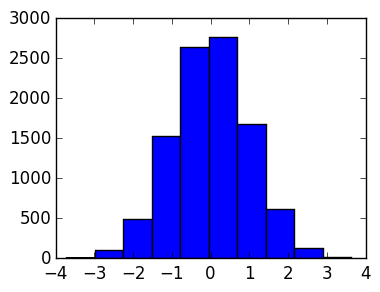We can check the distribution is correct by comparing it to a plot of the true distribution, using the normed=true option so that the bins are normalized to sum to one and the bins=... option to specify more refined bins:

In :
plt[:hist](randn(100000);bins=-5.:0.3:5.,normed=true);
x=-5.:0.1:5.
plot(x,exp(-x.^2/2)/sqrt(2π));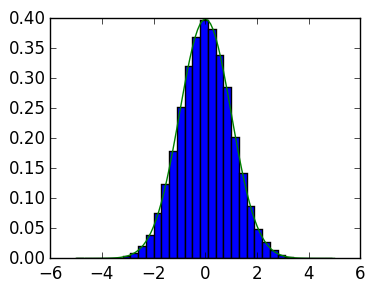Exercise 1(a) Plot a histogram for the numbers generated by rand. Can you guess the distribution?

In :
plt[:hist](rand(100000);normed=true);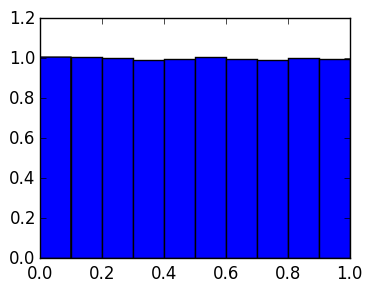Exercise 1(b) Create a function rande(ε,N) that returns N points of magnitude at most ε. Plot a histogram with ε=0.01 and n=10000 to verify your approach works.

In :
rande(ε,N)=ε*(2rand(N)-1)

ε=0.01
plt[:hist](rande(ε,10000));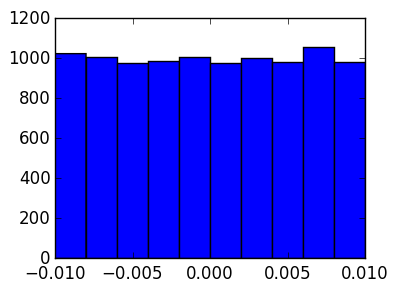## Condition numbers for scalar problems¶

Consider the problem $f : \mathbb R \rightarrow \mathbb R$ with the absolute value norm attached defined by:

$$f(x) = x^2$$

Recall the definition of absolute condition number

$$\hat \kappa_f( x, \epsilon) \triangleq \sup_{|\Delta x| \leq \epsilon} {|f( x + \Delta x) - f( x)| \over | \Delta x|}$$

and relative condition number

$$\kappa_f(x, \epsilon) \triangleq \sup_{|\Delta x| \leq \epsilon} {|f( x + \Delta x) - f( x)| \over |f( x)|} {| x| \over |\Delta x|}$$

Exercise 2(a) Use the random number generator from 1(b) to estimate the absolute condition number around $x=1$, and $\epsilon =0.01$, by finding the maximum value of

$${|f( x + \Delta x) - f( x)| \over | \Delta x|}$$

(Hint: maximum(v) returns the largest number in a vector v.) Ensure that your approximation is accurate by increasing the number of random points N used until you observe that the first two digits have converged.

In :
f(x)=x.^2
Δx=rande(0.01,1000)
x=1.
maximum(abs(f(x+Δx)-f(x))./abs(Δx))

Out:
2.009981633644511

Exercise 2(b) Repeat 2(a) with $x=2$ and $x=3$, and with $\epsilon =0.001$ and $\epsilon =0.0001$. Can you conjecture a formula for $\hat \kappa_f(x,0)$? (Advanced: Prove that this conjecture is true.)

In :
f(x)=x.^2
Δx=rande(0.01,1000)
x=2.
maximum(abs(f(x+Δx)-f(x))./abs(Δx))

Out:
4.0099899716156875
In :
f(x)=x.^2
Δx=rande(0.001,1000)
x=3.
maximum(abs(f(x+Δx)-f(x))./abs(Δx))

Out:
6.000996602725692

Exercise 2(c) Repeat parts 2(a) and 2(c) with the relative condition number. What formula do you conjecture for $\kappa_f(x,0)$? (Advanced: Prove that this conjecture is true.)

In :
f(x)=x.^2
Δx=rande(0.01,1000)
x=3.
maximum(abs(f(x+Δx)-f(x))./abs(Δx).*abs(x)./abs(f(x)))

Out:
2.0033304597086774

Exercise 2(d) Repeat parts 2(a-c) with $f(x)=x^3$. Conjecture a formula for the absolute and relative condition number when $f$ is a smooth function, in terms of the derivative of $f$. (Advanced: Prove that this conjecture is true.)

In :
f(x)=x.^3
Δx=rande(0.001,1000)
x=3.
maximum(abs(f(x+Δx)-f(x))./abs(Δx)),maximum(abs(f(x+Δx)-f(x))./abs(Δx).*abs(x)./abs(f(x)))

Out:
(27.008998265314982,3.00099980725722)

# Error analysis of scalar problems¶

We now consider the forward and backward error for floating point arithmetic. We will use BigFloat to simulate exact arithmetic. (This is an approximation, but the errors will be much smaller so is justified.) Recall that we are interested in the relative forward error

$${|f(x) - \tilde f(x)| \over |f(x)|}$$

and the relative backward error:

$$|\Delta x|$$

so that $\tilde f(x)=f(\Delta x)$.

The BigFloat(x) command converts a number to a BigFloat format, which is a floating point number with a lot more bits (default is 256 bits, though BigFloat can use even more if needed).

In :
BigFloat(0.25)

Out:
2.500000000000000000000000000000000000000000000000000000000000000000000000000000e-01

Warning Only convert numbers from Float64 if they are viewed as exact. The example below was fine, since 0.25 is representable exactly in Float64. The following shows issues converting 1/3:

In :
x64=1/3
x_wrong=BigFloat(x64)            # x is only accurate to 52 base-2 bits, so the bits are incorrect
x=BigFloat(1)/BigFloat(3)      # Both 1 and 3 are representable exactling as BigFloats

x_wrong,x  # the latter is accurate to last base-2 bit

Out:
(3.333333333333333148296162562473909929394721984863281250000000000000000000000000e-01,3.333333333333333333333333333333333333333333333333333333333333333333333333333348e-01)

Exercise 3(a) Calculate the relative forward error of approximating $f(x) = x^2$ by $\tilde f(x) = {\rm fl}(x) \otimes {\rm fl}(x)$ for $x=1/3$ in Float64 arithmetic, using BigFloat to approximate the true $f(x)$.

In :
abs(BigFloat(x64^2)-x^2)/abs(x^2)

Out:
5.551115123125782702118158340454101562500000000000000000000000755664748570763905e-17

Exercise 3(b) Calculate numerically the $\delta$ so that $\tilde f(x) = x^2(1+\delta)$. Verify that

x^2*(1+δ)-x64^2


is zero.

In :
δ=BigFloat(x64^2)/x^2-1

x^2*(1+δ)-x64^2

Out:
0.000000000000000000000000000000000000000000000000000000000000000000000000000000

Exercise 3(d) Calculate the backward error Δx of approximate $f$ by $\tilde f$ at $x = 1/3$. Verify that

(x+Δx)^2 - x64^2


is zero (to roughly 75 digits).

In :
Δx=x*sqrt(1+δ)-x
println(abs(Δx))

(x+Δx)^2-x64^2

9.251858538542971298592260193239229704918142961922806396793759981798853374656124e-18

Out:
-2.159042138773611156346587965700099892779000091109070346255925867542147950790607e-78

## Conditioning of Householder reflections¶

Last lab we considered the Householder reflection constructed from a vector $\mathbf x$ via:

\begin{align*} \mathbf w &= \mathbf x - \alpha \|{\mathbf x}\| \mathbf e_1, \qquad \alpha = \pm 1,\cr \mathbf v &= \mathbf w / \|\mathbf w\|,\cr Q_{\mathbf v} &\triangleq I - 2 \mathbf v \mathbf v^\top \end{align*}

so that

$$Q_{\mathbf v}\mathbf x = \begin{pmatrix} \alpha \|\mathbf x\| \cr 0 \cr \vdots \cr 0\end{pmatrix}$$

An oustanding question is the choice of $\alpha$: $\alpha = {\rm sign}(x_1)$ or $\alpha = -{\rm sign}(x_1)$. We will investigate this question by looking at the error of the problem

$$f(\mathbf x) = Q_{\mathbf v} \mathbf x$$

where we know the exact value, versus the implementation on a computer in floating point arithmetic, which we denote $\tilde f(\mathbf x)$. Here we have $f, \tilde f : \mathbb R^n \rightarrow \mathbb R^n$ with the 2-norm attached.

Exercise 4(a) Write a function house(s,x) that returns $Q_{\mathbf v}$ as definded above, with \alpha = s*sign(x).

In :
function house(s,x)
n=length(x)
α=s*sign(x)
v=x-α*norm(x)*[1;zeros(n-1)]
v=v/norm(v)
I-2v*v'
end

Out:
house (generic function with 1 method)

Exercise 4(b) Consider the vector $\mathbf x=[1.,\epsilon]$. Plot the forward error of $\|f(\mathbf x)-\tilde f(\mathbf x)\|$ for s=1. (Hint: use a loglog plot with ε = 1./logspace(1,13,100).)

In :
ε=1./logspace(1,13,100)
err=Array(Float64,length(ε))

for k=1:length(ε)
x=[1.,ε[k]]
err[k]=norm(house(1,x)*x-[norm(x),0.])
end

loglog(ε,err);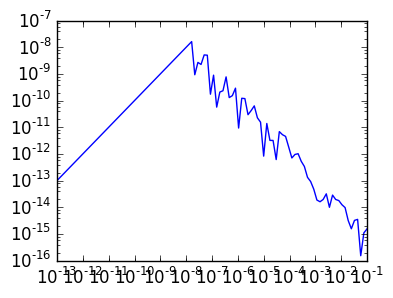4(c) Repeat the previous exercise with and s=-1. What do you conclude about the stability of the two choices?

In :
ε=1./logspace(1,13,100)
err=Array(Float64,length(ε))

for k=1:length(ε)
x=[1.,ε[k]]
err[k]=norm(house(-1,x)*x-[-norm(x),0.])
end

loglog(ε,err);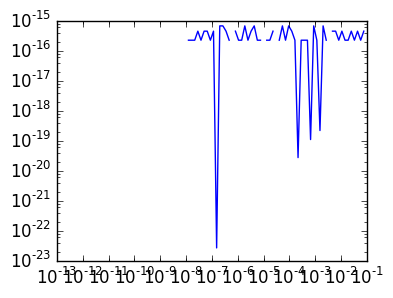3(d) Backward error analysis for this problem is impossible. Why is that?